Question

While preparing for their comeback tour, The Flaming Rogers find that the average time it takes their sound tech to set up for a show is 56.1 minutes, with a standard deviation of 6.4 minutes. If the band manager decides to include only the fastest 23% of sound techs on the tour, what should the cutoff time be for concert setup? Assume the times are normally distributed.

1.thanhha

The cutoff time be for concert setup should be of 51.4 minutes.

Step-by-step explanation:

Normal Probability Distribution:

Problems of normal distributions can be solved using the z-score formula.

In a set with mean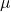and standard deviation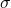, the z-score of a measure X is given by: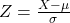The Z-score measures how many standard deviations the measure is from the mean. After finding the Z-score, we look at the z-score table and find the p-value associated with this z-score. This p-value is the probability that the value of the measure is smaller than X, that is, the percentile of X. Subtracting 1 by the p-value, we get the probability that the value of the measure is greater than X.

Average time it takes their sound tech to set up for a show is 56.1 minutes, with a standard deviation of 6.4 minutes.

This means that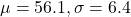If the band manager decides to include only the fastest 23% of sound techs on the tour, what should the cutoff time be for concert setup?

The cutoff time would be the 23rd percentile of times, which is X when Z has a p-value of 0.23, so X when Z = -0.74.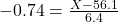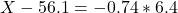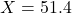The cutoff time be for concert setup should be of 51.4 minutes.